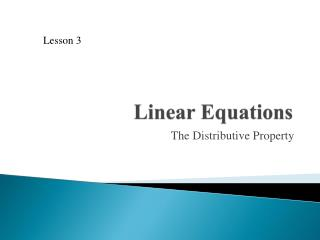DownloadDownload PresentationLinear Equations

# Linear Equations

Télécharger la présentation## Linear Equations

- - - - - - - - - - - - - - - - - - - - - - - - - - - E N D - - - - - - - - - - - - - - - - - - - - - - - - - - -
##### Presentation Transcript

1. Lesson 3 Linear Equations The Distributive Property

2. Warm-Up State whether the equation is true or not with the given values. • 6x – 4y = 22 when x = 5 and y = 2 • x + 5 = y when x = 6 and y = 11 • y = – 3x – 1 when x = – 2 and y = – 7

3. The Distributive Property Target: Simplify expressions using the Distributive Property and combining like terms.

4. Vocabulary • Term: A number or the product of a number and a variable. • Constant: Term that has no variable. • Coefficient: The number multiplied by a variable in a term. • Distributive Property: A property that can be used to rewrite an expression without parentheses. • Like Terms: Terms that have the same variable raised to the same power. • Equivalent Expressions: Expressions that have the same value.

5. The Distributive Property For any numbers a, b and c: a(b + c) = a ∙ b + a ∙ c a(b – c) = a ∙ b – a ∙ c

6. Example 1a Use the Distributive Property to simplify each expression. 2(x + 6) 2(x) + 2(6) 2x + 12

7. Example 1b Use the Distributive Property to simplify each expression.

8. Example 1c Use the Distributive Property to simplify each expression. – 5(3x – 1) – 5(3x) – (– 5)(1) – 15x + 5

9. Example 2a Find the product by using the Distributive Property and mental math. 4(103) 4(100 + 3) 4(100) + 4(3) 400 + 12 412

10. Example 2b Find the product by using the Distributive Property and mental math. 998 ∙ 7 7(1000 – 2) 7(1000) – 7(2) 7000 – 14 6986

11. Example 2c Find the product by using the Distributive Property and mental math. 8(6.5) 8(6 + 0.5) 8(6) + 8(0.5) 48 + 4 52

12. Example 3 Simplify by combining like terms. 3x – 2y + 4 – 2x + x + 4y 2x + 2y + 4

13. Example 4 Simplify by combining like terms – 5(2x – 1) + 3x – 2 – 10x + 5 + 3x – 2 – 7x + 3

14. Exit Problems Simplify each expression. • 4(x − 3) • 9x + 2 + 3x – 4 − 7x • 10 + 8(2x + 3) − x

15. Communication Prompt How could the Distributive Property help you do mental math in real-world situations?